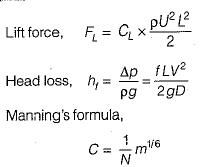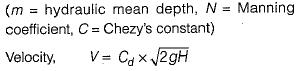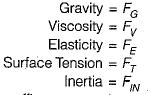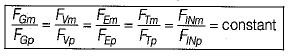Courses

# Test: Dimensional & Model Analysis - 1

## 10 Questions MCQ Test Fluid Mechanics | Test: Dimensional & Model Analysis - 1

Description
This mock test of Test: Dimensional & Model Analysis - 1 for Mechanical Engineering helps you for every Mechanical Engineering entrance exam. This contains 10 Multiple Choice Questions for Mechanical Engineering Test: Dimensional & Model Analysis - 1 (mcq) to study with solutions a complete question bank. The solved questions answers in this Test: Dimensional & Model Analysis - 1 quiz give you a good mix of easy questions and tough questions. Mechanical Engineering students definitely take this Test: Dimensional & Model Analysis - 1 exercise for a better result in the exam. You can find other Test: Dimensional & Model Analysis - 1 extra questions, long questions & short questions for Mechanical Engineering on EduRev as well by searching above.
QUESTION: 1

### Dimensional analysis is useful in Dimensional analysis deals with the process whereby all the important factors involved in a physical phenomenon are systematically arranged into dimensional group.

Solution:

Dimensional analysis deals with the process whereby all the important factors involved in a physical phenomenon are systematically arranged into dimensional group.

QUESTION: 2

Solution:
QUESTION: 3

### A 1 : 20 model of a spillw ay dissipated 0.25 hp. The corresponding prototype horsepower dissipated will, be

Solution:
QUESTION: 4

Which of the following is not a dimensionless number?

Solution:QUESTION: 5

Dynamic similarity between model and prototype implies that

Solution:

Two systems are dynamically similar , if geometric and kinematic similarities exist and further the ratios of all corresponding forces in the two systems are the same.
If forces due toand suffixees m and p stand for model and prototype respectively, strict dynamic similarity means:QUESTION: 6

Principles of similitude form the basis of

Solution:
QUESTION: 7

Reynolds model law is applicable for

Solution:
QUESTION: 8

The Froude’s model law would not be applicable for the analysis of

Solution:
QUESTION: 9

Mach number is significant in

Solution:
QUESTION: 10

Weber number will be an important consideration in the study of

Solution: